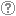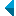# Search the repositoryQuery: search in TitleAuthorAbstractKeywordsFull textYear of publishing ANDORAND NOT search in TitleAuthorAbstractKeywordsFull textYear of publishing ANDORAND NOT search in TitleAuthorAbstractKeywordsFull textYear of publishing ANDORAND NOT search in TitleAuthorAbstractKeywordsFull textYear of publishing Work type: All work types Habilitation (m4) Specialist thesis (m3) High school thesis (m6) Bachelor work * (dip) Master disertations * (mag) Doctorate disertations * (dok) Research Data or Corpuses (data) * old and bologna study programme Language: All languagesSlovenianEnglishGermanCroatianSerbianBosnianBulgarianCzechFinnishFrenchGerman (Austria)HungarianItalianJapaneseLithuanianNorwegianPolishRussianSerbian (cyrillic)SlovakSpanishSwedishTurkishUnknown Search in: RUP    FAMNIT - Faculty of Mathematics, Science and Information Technologies    FHŠ - Faculty of Humanities    FM - Faculty of Management    FTŠ Turistica - Turistica – College of Tourism Portorož    FVZ - Faculty of Health Sciences    IAM - Andrej Marušič Institute    PEF - Faculty of Education    UPR - University of Primorska    ZUP - University of Primorska PressCOBISS    Fakulteta za humanistične študije, Koper    Fakulteta za management Koper in Pedagoška fakulteta Koper    Fakulteta za vede o zdravju, Izola    Knjižnica za tehniko, medicino in naravoslovje, Koper    Turistica, Portorož    Znanstveno-raziskovalno središče Koper Options: Show only hits with full text Reset

 1 - 5 / 511.On the Terwilliger algebra of bipartite distance-regular graphs with [Delta][sub]2 = 0 and c[sub]2=1Štefko Miklavič, Mark MacLean, Safet Penjić, 2016, original scientific articleAbstract: Let ▫$\Gamma$▫ denote a bipartite distance-regular graph with diameter ▫$D \geq 4$▫ and valency ▫$k \geq 3$▫. Let ▫$X$▫ denote the vertex set of ▫$\Gamma$▫, and let ▫$A$▫ denote the adjacency matrix of ▫$\Gamma$▫. For ▫$x \in X$▫ and for ▫$0 \leq i \leq D$▫, let ▫$\operatorname{\Gamma}_i(x)$▫ denote the set of vertices in ▫$X$▫ that are distance ▫$i$▫ from vertex ▫$x$▫. Define a parameter ▫$\operatorname{\Delta}_2$▫ in terms of the intersection numbers by ▫$\operatorname{\Delta}_2 = (k - 2)(c_3 - 1) -(c_2 - 1) p_{22}^2$▫. We first show that ▫$\operatorname{\Delta}_2 = 0$▫ implies that ▫$D \leq 5$▫ or ▫$c_2 \in \{1, 2 \}$▫. For ▫$x \in X$▫ let ▫$T = T(x)$▫ denote the subalgebra of ▫$\text{Mat}_X(\mathbb{C})$▫ generated by ▫$A, E_0^\ast, E_1^\ast, \ldots, E_D^\ast$▫, where for ▫$0 \leq i \leq D$, $E_i^\ast$▫ represents the projection onto the▫ $i$▫th subconstituent of ▫$\Gamma$▫ with respect to ▫$x$▫. We refer to ▫$T$▫ as the Terwilliger algebra of ▫$\Gamma$▫ with respect to ▫$x$▫. By the endpoint of an irreducible ▫$T$▫-module ▫$W$▫ we mean ▫$\min \{i | E_i^\ast W \ne 0 \}$▫. In this paper we assume ▫$\Gamma$▫ has the property that for ▫$2 \leq i \leq D - 1$▫, there exist complex scalars ▫$\alpha_i$▫, ▫$\beta_i$▫ such that for all ▫$x, y, z \in X$▫ with ▫$\partial(x, y) = 2$▫, ▫$\partial(x, z) = i$▫, ▫$\partial(y, z) = i$▫, we have ▫$\alpha_i + \beta_i | \operatorname{\Gamma}_1(x) \cap \operatorname{\Gamma}_1(y) \cap \operatorname{\Gamma}_{i - 1}(z) | = | \operatorname{\Gamma}_{i - 1}(x) \cap \operatorname{\Gamma}_{i - 1}(y) \cap \operatorname{\Gamma}_1(z) |$▫. We additionally assume that▫ $\operatorname{\Delta}_2 = 0$▫ with ▫$c_2 = 1$▫. Under the above assumptions we study the algebra ▫$T$▫. We show that if ▫$\Gamma$▫ is not almost 2-homogeneous, then up to isomorphism there exists exactly one irreducible ▫$T$▫-module with endpoint 2. We give an orthogonal basis for this ▫$T$▫-module, and we give the action of ▫$A$▫ on this basis.Found in: osebiKeywords: distance-regular graphs, terwilliger algebra, subconstituent algebraPublished: 14.11.2017; Views: 1662; Downloads: 130Full text (0,00 KB) 2.An A-invariant subspace for bipartite distance-regular graphs with exactly two irreducible T-modules with endpoint 2, both thinŠtefko Miklavič, Mark MacLean, Safet Penjić, 2018, original scientific articleFound in: osebiKeywords: distance-regular graph, Terwilliger algebra, subconstituent algebra, A-invariant subspacePublished: 02.03.2018; Views: 1478; Downloads: 80Full text (0,00 KB) 3.Bipartite distance-regular graphs and taut pairs of pseudo primitive idempotentsŠtefko Miklavič, Mark MacLean, 2019, original scientific articleFound in: osebiKeywords: distance-regular graph, pseudo primitive idempotent, taut pairPublished: 20.08.2019; Views: 1180; Downloads: 147Full text (0,00 KB) 4.On a certain class of 1-thin distance-regular graphsŠtefko Miklavič, Mark MacLean, 2020, original scientific articleFound in: osebiKeywords: distance-regular graph, Terwilliger algebra, subconstituent algebraPublished: 20.07.2020; Views: 802; Downloads: 83This document has more files! More... 5.A combinatorial basis for Terwilliger algebra modules of a bipartite distance-regular graphMark MacLean, Safet Penjić, 2021, original scientific articleFound in: osebiKeywords: bipartite distance-regular graph, Terwilliger algebra, subconstituent algebra, T-modules of endpoint 2Published: 06.05.2021; Views: 299; Downloads: 26Full text (0,00 KB)
Search done in 0 sec.